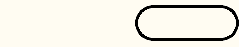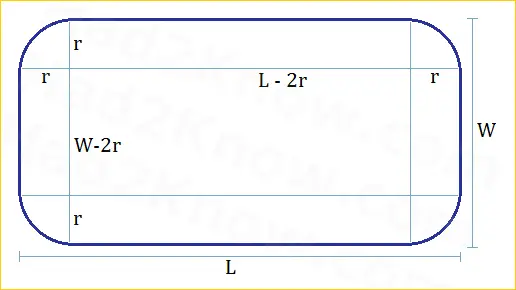# Perimeter and Area of a Rounded Rectangle

Rounded Rectangle Calculator
W =
L =
r =

Rounded rectangles have corners that are smoothed out with quarter-circle arcs. Rectangles that have rounded corners instead of square corners are pleasing to the eye and are frequently used in construction and design. You can find examples in home décor, furniture, landscaping designs, and other industrial applications.

Oval-shaped racetracks (rectangles with semicircles appended to opposite sides) are related to rounded rectangles:The area and perimeter of a rounded rectangle depends on the overall width (W) and length (L) of the shape as well as the radius (r) of curvature at the corners. If you know the three measurements, you can find the perimeter and area using the formulas below or the calculator on the left.The area formula in terms of W, L, and r is

Area = LW - 4r2 + πr2
= LW - (4-π)r2

The perimeter formula in terms of W, L, and r is

Perimeter = 2L + 2W - 8r + 2πr
= 2L + 2W - (8-2π)r

Example: A rounded square has a total width and length of 12 inches. The radius of curvature at the rounded corners is 2.5 inches. What is the area and perimeter of the shape?

In this example we have W = L = 12 and r = 2.5. The area and perimeter are calculated by

Area = 12*12 - (4-π)2.5*2.5
= 138.635 square inches

Perimeter = 2*12 + 2*12 - (8-2π)2.5
= 43.708 inches.